# 相关库文件

pip install requests


# 编码过程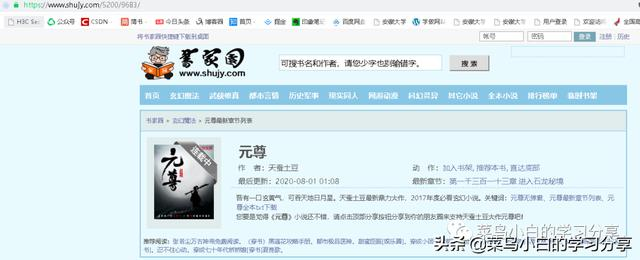import requests
url ='https://www.shujy.com/5200/9683/'
response = requests.get(url)
html = response.text

print(html)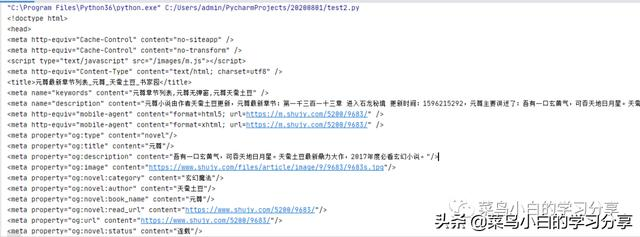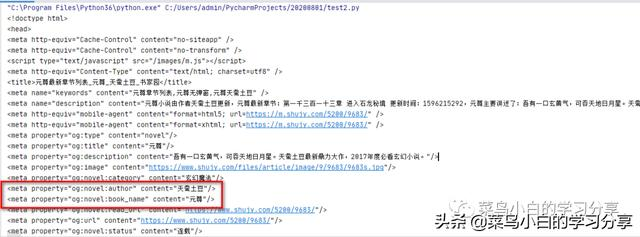title = re.findall(r'<meta property="og:novel:book_name" content="(.*?)"/>',html)
author = re.findall(r'<meta property="og:novel:author" content="(.*?)"/>',html)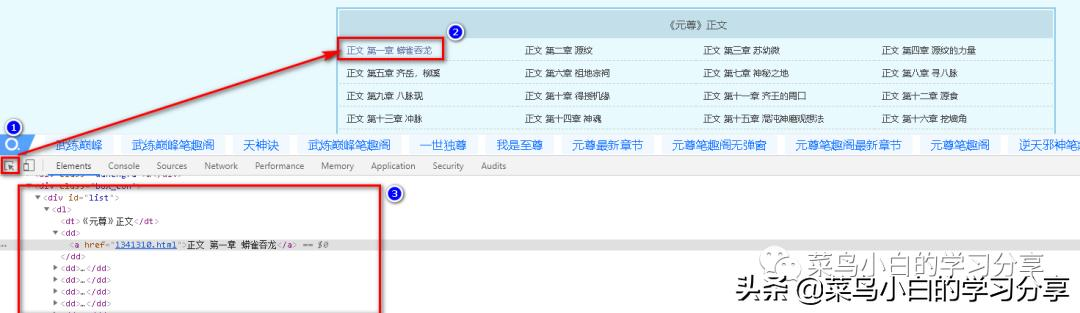1. 点击左上角的箭头
2. 点击我们需要定位的元素，我们需要找到每一章的链接，所以我们点击“正文 第一章”
3. 我们就可以看到开发者工具中出现了对象在html中的位置

dl =re.findall(r'<div id="list">.*?</div>',html,re.S)
chapter_info_list=re.findall(r'<a href="(.*?)">(.*?)</a>',dl)


chapter_url = url+'/'+chapter_url
chapter_url = chapter_url.replace(' ','')


chapter_response = requests.get(chapter_url)
chapter_html = chapter_response.text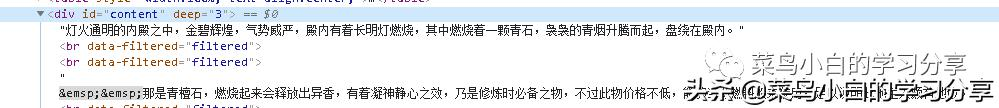#获取第一页正文内容
chapter_content = re.findall(r'<div id="content">(.*?)</div>',chapter_html,re.S)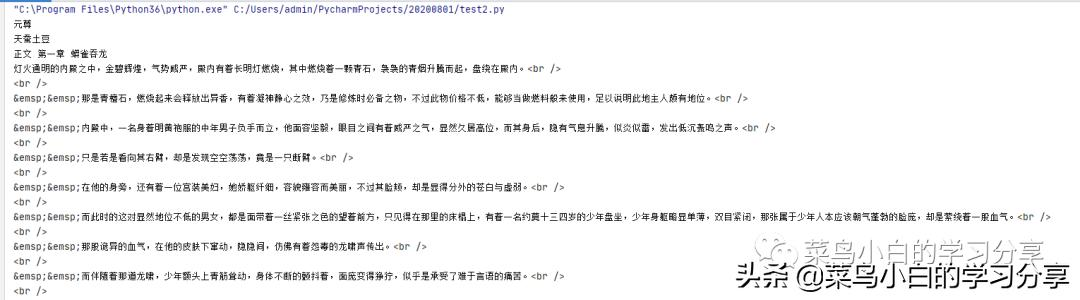chapter_content = chapter_content.replace('&emsp;&emsp;','')
chapter_content = chapter_content.replace('<br />','')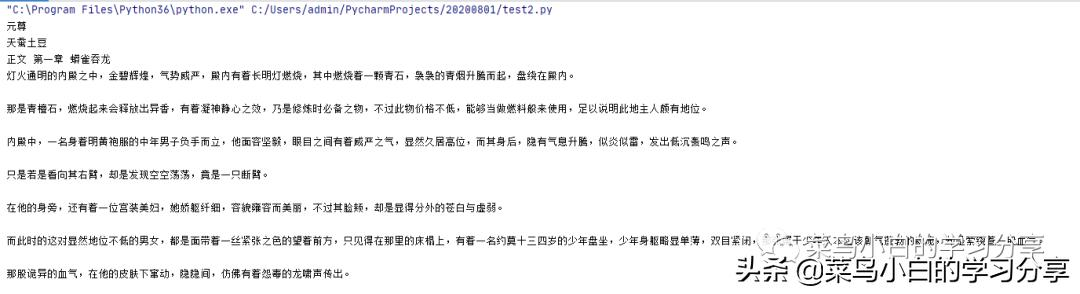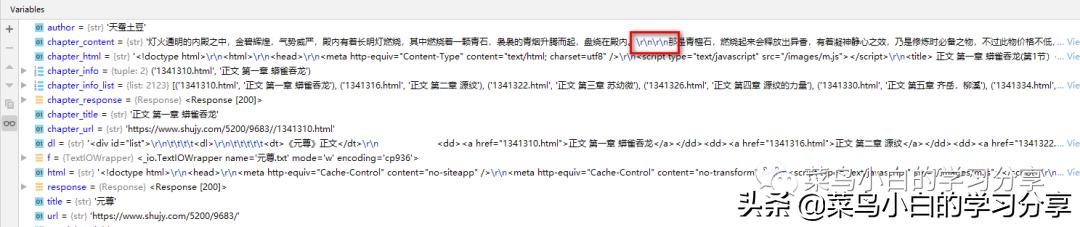chapter_content = chapter_content.replace('\r\n\r', '')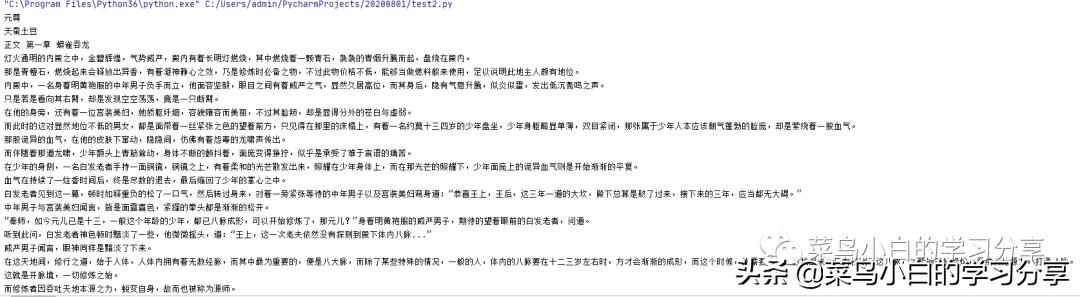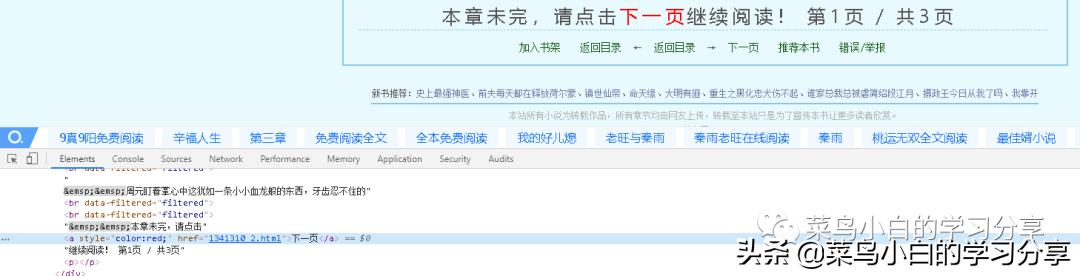chapter_url,current_page,total_pages = re.findall(r'本章未完，请点击<a style="color:red;" href="(.*?)">下一页</a>继续阅读！第(.*?)页 / 共(.*?)页</p>', chapter_content,re.S)


for i in range(1,int(total_pages)):
chapter_url,current_page,total_pages = re.findall(r'本章未完，请点击<a style="color:red;" href="(.*?)">下一页</a>继续阅读！第(.*?)页 / 共(.*?)页</p>',chapter_content)
chapter_url = url+'/'+chapter_url
chapter_url = chapter_url.replace(' ','')
chapter_response = requests.get(chapter_url)
chapter_html =chapter_response.text
chapter_content = re.findall(r'<div id="content">(.*?)</div>', chapter_html, re.S)
chapter_content = chapter_content.replace('&emsp;&emsp;', '')
chapter_content = chapter_content.replace('<br />', '')
chapter_content = chapter_content.replace('\r\n\r', '')
f.write('\n')
f.write(chapter_content)


with open('%s.txt'%title,'w') as f:
f.write(title)
f.write('\n')
f.write(author)
f.write('\n')


# list index out of range报错的处理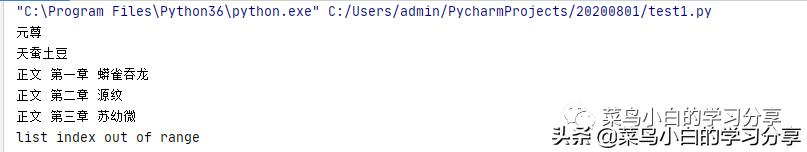1. list[index]index超出范围
2. list是一个空的，没有一个元素，进行list就会出现错误！

def find(pattern,string,url):
try:
chapter_content = re.findall(pattern, string, re.S)
return chapter_content
except Exception as e:
print(e)
time.sleep(1)
chapter_response = requests.get(url)

chapter_html = chapter_response.text
print(chapter_html)
print(url)
i = find(pattern,chapter_html,url)
return i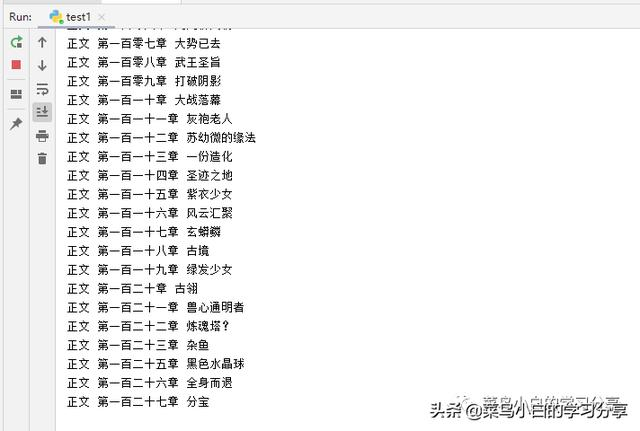posted @ 2020-08-01 22:34  菜鸟小白的学习分享  阅读(43)  评论(0编辑  收藏